### Showing videos fromEddie Woo with a total of 4,051 videos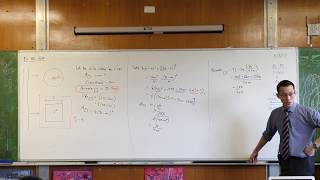04:31
Max/Min Question: Cutting a Wire in Two (2 of 2: Finding the minimum)
1y9m ago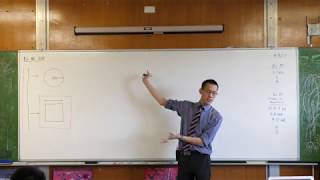10:08
Max/Min Question: Cutting a Wire in Two (1 of 2: Setting up the equations)
1y9m ago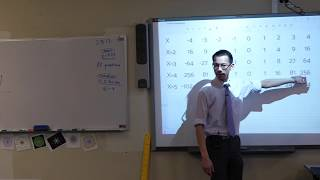06:01
The Power Curves (2 of 2: Considering higher powers)
1y9m ago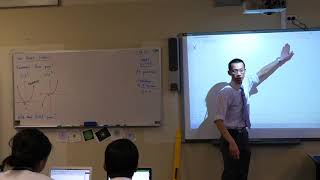07:20
The Power Curves (1 of 2: Investigating through tables of values)
1y9m ago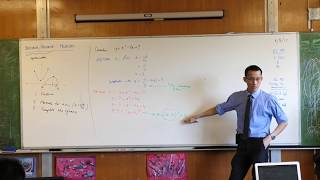05:47
Maximum/Minimum with Quadratics (2 of 2: Completing the square)
1y9m ago08:30
Maximum/Minimum with Quadratics (1 of 2: Axis of symmetry)
1y9m ago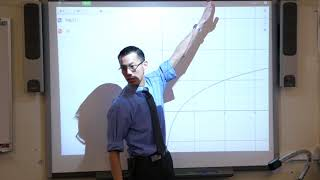10:08
Graphs of Logarithms (3 of 3: Visualising the shape)
1y9m ago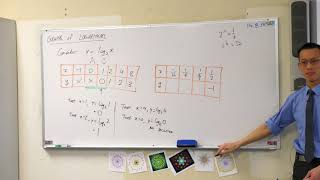09:16
Graphs of Logarithms (2 of 3: Investigating near x=0)
1y9m ago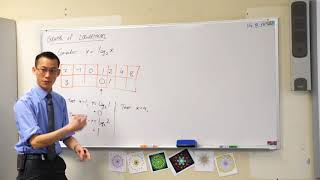07:59
Graphs of Logarithms (1 of 3: Considering integer values)
1y9m ago08:37
Equations Reducible to Quadratics (Changing Logarithm Bases)
1y9m ago05:26
Solving Equations with Radical Functions (2 of 2: Final solution)
1y9m ago10:58
Solving Equations with Radical Functions (1 of 2: Initial steps & discussion)
1y9m ago10:00
Equation of a Parabola - Vertex Form (Completing the square)
1y9m ago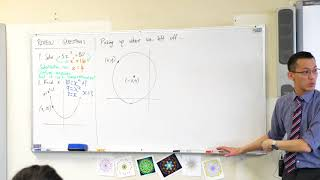06:10
Y10 Review Questions (Solving quadratic equations)
1y9m ago11:40
Proof by Contradiction (2 of 2: Infinite primes)
1y9m ago08:34
Proof by Contradiction (1 of 2: How does it work?)
1y9m ago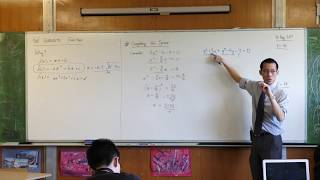06:58
Introduction to Quadratic Theory (2 of 2: Completing the square)
1y9m ago06:45
1y9m ago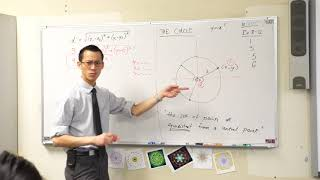06:42
The Circle (2 of 2: Constructing the Cartesian equation)
1y9m ago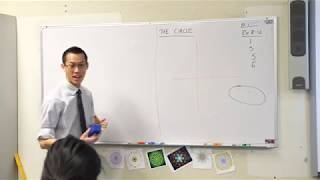07:34
The Circle (1 of 2: Starting with a verbal definition)
1y9m ago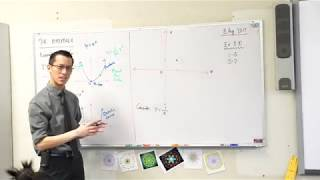13:20
The Hyperbola (2 of 2: Understanding the graph & its behaviour)
1y9m ago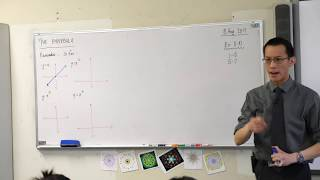10:19
The Hyperbola (1 of 2: Review of earlier graphs)
1y9m ago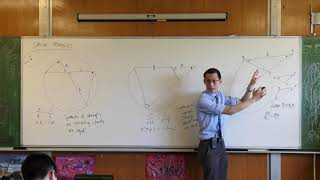12:28
Intercept Properties - Circle Geometry (3 of 3: Tangent & secant)
1y9m ago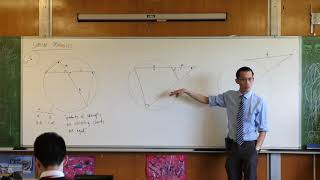05:14
Intercept Properties - Circle Geometry (2 of 3: Secants from a point)
1y9m ago05:59
Intercept Properties - Circle Geometry (1 of 3: Intersecting chords)
1y9m ago09:53
Angle in the Alternate Segment - Circle Geometry (3 of 3: Conclusion and overall summary)
1y9m ago09:39
Angle in the Alternate Segment - Circle Geometry (2 of 3: Proof)
1y9m ago07:15
Angle in the Alternate Segment - Circle Geometry (1 of 3: Setup)
1y9m ago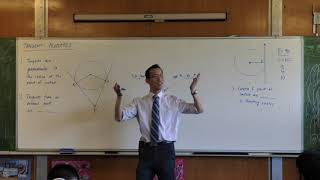09:21
Tangent Properties - Circle Geometry (Tangents from an external point are equal)
1y9m ago09:21
Tangent Properties - Circle Geometry (Tangent is perpendicular to radius)
1y9m ago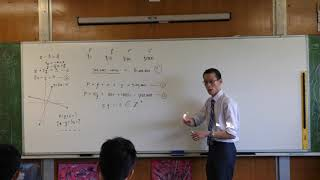06:53
Enrichment Problem: \$1,000,000 in Specific Denominations (2 of 2: Solution)
1y9m ago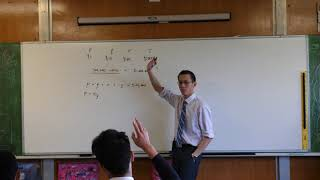08:19
Enrichment Problem: \$1,000,000 in Specific Denominations (1 of 2: Setup)
1y9m ago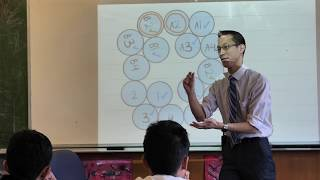08:40
Enrichment Problem: Arranging Counters on a Plane
1y9m ago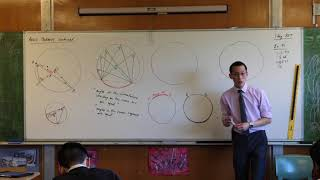10:19
Angle Properties - Circle Geometry (Angles in the same segment)
1y9m ago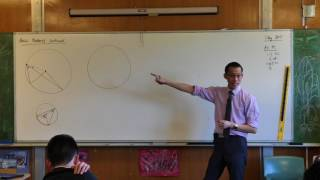07:21
Angle Properties - Circle Geometry (Second proof of angle at the centre/circumference)
1y9m ago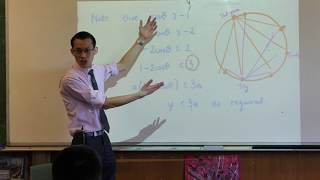08:37
HSC Geometry Question (2 of 2: Proving the inequality)
1y9m ago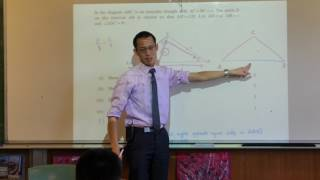07:50
HSC Geometry Question (1 of 2: Proving & using triangle similarity)
1y9m ago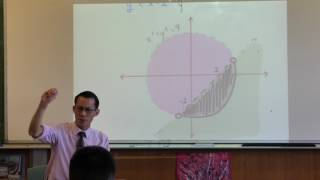11:12
Prelim Mathematics Ext 1 Quiz (Inequality regions, solving trigonometric equations)
1y9m ago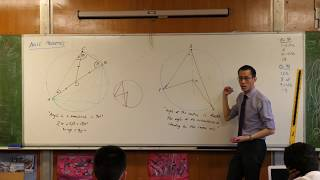07:19
Angle Properties - Circle Geometry (Angle at the centre/circumference)
1y9m ago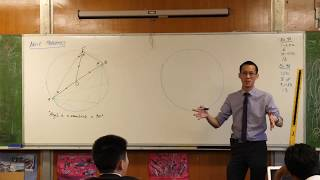09:40
Angle Properties - Circle Geometry (Angle in a semicircle)
1y9m ago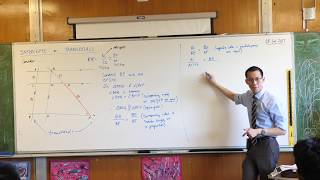08:34
Ratios of Intercepts on Transversals (2 of 2: Constructing the proof)
1y9m ago11:37
Ratios of Intercepts on Transversals (1 of 2: Setting up the problem)
1y9m ago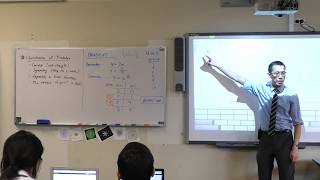11:20
Introduction to Parabolas (2 of 2: Characteristics & translations)
1y9m ago10:34
Introduction to Parabolas (1 of 2: Investigating the shape)
1y9m ago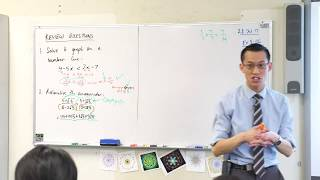07:29
Y10 Review Questions (Solving an inequality, rationalising a denominator)
1y9m ago09:38
Inequality Proofs (Example 5 of 5: Investigating a large product)
1y9m ago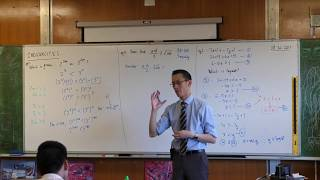05:25
Inequality Proofs (Example 4 of 5: Arithmetic & Geometric Means)
1y9m ago06:15
Inequality Proofs (Example 3 of 5: Is 2^300 bigger or 3^200?)
1y9m ago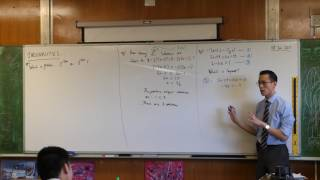05:42
Inequality Proofs (Example 2 of 5: Which number is largest?)
1y10m ago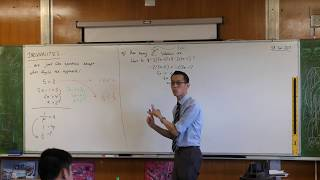11:07
Inequality Proofs (Example 1 of 5: How many positive integer solutions?)
1y10m ago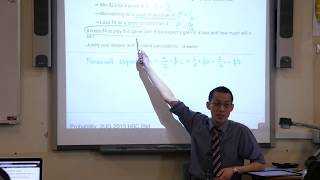09:14
Financial Expectation (2 of 2: When the game isn't free)
1y10m ago12:53
Quadrilaterals (Relationships, Properties & Sufficiency Conditions)
1y10m ago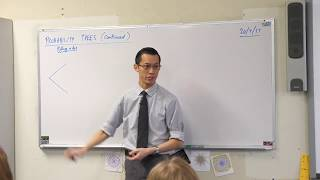07:36
Probability Trees (1 of 2: How to construct them)
1y10m ago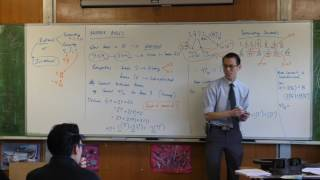08:02
Number Bases (5 of 5: Conversion to binary & hexadecimal)
1y10m ago06:49
Number Bases (4 of 5: Converting between bases)
1y10m ago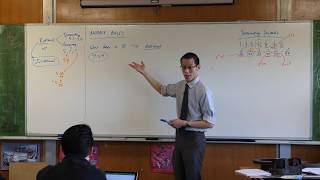07:10
Number Bases (3 of 5: What is the "base" of a number system?)
1y10m ago08:36
Number Bases (2 of 5: Investigating decimal expansions)
1y10m ago10:15
Number Bases (1 of 5: Exploring numbers through a spreadsheet)
1y10m ago